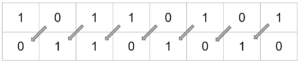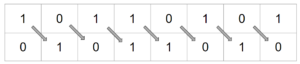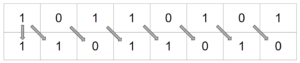# Binary Shifts

## Intro

### Binary Shifts

https://youtu.be/G-9fwJbgRjA

## Logical Shifts

### Logical Left ShiftIn a left shift each bit is simply moved to the left with the empty space on the right replaced with zero.

Example

000110
<< 2
011000

Notice the ones have moved along 2 spaces

### Logical Right ShiftIn a logical right shift the bits are shifted to the right and the most significant bit(empty bit to the left ) is replace with zero. The least significant bit(Any bit falling off the right) is discarded

Example

```0011001
>>1
0001100```

Some programming languages don’t have an in-built logical right shift, they only have an arithmetic right shift.

## Arithmetic Shifts

###Arithmetic left shifts work the same as logical left shifts. The bit is shifted to the left (the sign bit is discarded) with zeroes add at the right hand end.

Example

000110
<< 2
011000

### Arithmetic Right ShiftIn an arithmetic right shift the bit is shifted to the right but the most significant bit is copied to the next most significant bit position on the left.

This is used when the most significant bit is the sign bit (1s/2s Compliment) indicating + / – value. The least significant bit is discarded.

Example 1

```1011
>>1
1101```

Example 2

10110100
>>3
11110110

## Cyclic Shift

### Cyclic ShiftThese shifts can be performed either left or right. No data is discarded as the bit that falls off one end is added to the other end.

Example

```10110011
Shift 3 Right
01110110

```